# AP Calculus BC Practice Test 7

### Test Information9 questions18 minutes

Calculator Disallowed

1.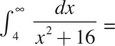2. What is the equation of the line tangent to the graph of y = sin2 x at x =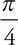?

3.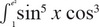x dx =

4. Evaluate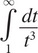5. If f(x) =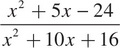, then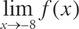is

6. What is the approximation of the value of e3 obtained by using the fourth-degree Taylor polynomial about x = 0 for ex?

7. A rock is thrown straight upward with an initial velocity of 50 m/s from a point 100 m above the ground. If the acceleration of the rock at any time t is a = -10 m/s2, what is the maximum height of the rock (in meters)?

8. The sum of the infinite geometric series 2 -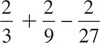+…is

9. What are all values of x for which the series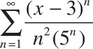converges?